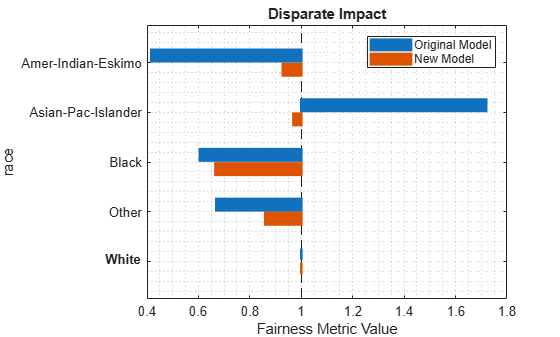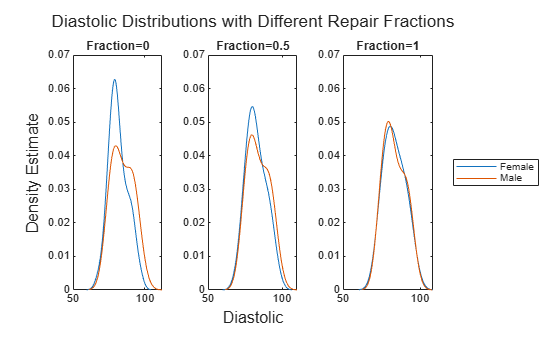# transform

Transform new predictor data to remove disparate impact

Since R2022b

## Syntax

``transformedData = transform(remover,Tbl)``
``transformedData = transform(remover,X,attribute)``
``transformedData = transform(___,Name=Value)``

## Description

example

````transformedData = transform(remover,Tbl)` transforms the predictor data in `Tbl` according to the transformation in the `disparateImpactRemover` object (`remover`). The predictor variables and sensitive attribute in `Tbl` must have the same names as the variables used to create `remover`. To see the variable names, use `remover.PredictorNames` and `remover.SensitiveAttribute`.To see the fraction of the data transformation used to return `transformedData`, use `remover.RepairFraction`.```
````transformedData = transform(remover,X,attribute)` returns the data `X`, transformed with respect to the sensitive attribute `attribute`.```

example

````transformedData = transform(___,Name=Value)` specifies options using one or more name-value arguments in addition to any of the input argument combinations in previous syntaxes. For example, you can specify the extent of the data transformation by using the `RepairFraction` name-value argument. A value of 1 indicates a full transformation, and a value of 0 indicates no transformation.```

## Examples

collapse all

Train a binary classifier, classify test data using the model, and compute the disparate impact for each group in the sensitive attribute. To reduce the disparate impact values, use `disparateImpactRemover`, and then retrain the binary classifier. Transform the test data set, reclassify the observations, and compute the disparate impact values.

Load the sample data `census1994`, which contains the training data `adultdata` and the test data `adulttest`. The data sets consist of demographic information from the US Census Bureau that can be used to predict whether an individual makes over \$50,000 per year. Preview the first few rows of the training data set.

```load census1994 head(adultdata)```
``` age workClass fnlwgt education education_num marital_status occupation relationship race sex capital_gain capital_loss hours_per_week native_country salary ___ ________________ __________ _________ _____________ _____________________ _________________ _____________ _____ ______ ____________ ____________ ______________ ______________ ______ 39 State-gov 77516 Bachelors 13 Never-married Adm-clerical Not-in-family White Male 2174 0 40 United-States <=50K 50 Self-emp-not-inc 83311 Bachelors 13 Married-civ-spouse Exec-managerial Husband White Male 0 0 13 United-States <=50K 38 Private 2.1565e+05 HS-grad 9 Divorced Handlers-cleaners Not-in-family White Male 0 0 40 United-States <=50K 53 Private 2.3472e+05 11th 7 Married-civ-spouse Handlers-cleaners Husband Black Male 0 0 40 United-States <=50K 28 Private 3.3841e+05 Bachelors 13 Married-civ-spouse Prof-specialty Wife Black Female 0 0 40 Cuba <=50K 37 Private 2.8458e+05 Masters 14 Married-civ-spouse Exec-managerial Wife White Female 0 0 40 United-States <=50K 49 Private 1.6019e+05 9th 5 Married-spouse-absent Other-service Not-in-family Black Female 0 0 16 Jamaica <=50K 52 Self-emp-not-inc 2.0964e+05 HS-grad 9 Married-civ-spouse Exec-managerial Husband White Male 0 0 45 United-States >50K ```

Each row contains the demographic information for one adult. The last column `salary` shows whether a person has a salary less than or equal to \$50,000 per year or greater than \$50,000 per year.

Remove observations from `adultdata` and `adulttest` that contain missing values.

```adultdata = rmmissing(adultdata); adulttest = rmmissing(adulttest);```

Specify the continuous numeric predictors to use for model training.

```predictors = ["age","education_num","capital_gain","capital_loss", ... "hours_per_week"];```

Train an ensemble classifier using the training set `adultdata`. Specify `salary` as the response variable and `fnlwgt` as the observation weights. Because the training set is imbalanced, use the `RUSBoost` algorithm. After training the model, predict the salary (class label) of the observations in the test set `adulttest`.

```rng("default") % For reproducibility mdl = fitcensemble(adultdata,"salary",Weights="fnlwgt", ... PredictorNames=predictors,Method="RUSBoost"); labels = predict(mdl,adulttest);```

Transform the training set predictors by using the `race` sensitive attribute.

```[remover,newadultdata] = disparateImpactRemover(adultdata, ... "race",PredictorNames=predictors); remover```
```remover = disparateImpactRemover with properties: RepairFraction: 1 PredictorNames: {'age' 'education_num' 'capital_gain' 'capital_loss' 'hours_per_week'} SensitiveAttribute: 'race' ```

`remover` is a `disparateImpactRemover` object, which contains the transformation of the `remover.PredictorNames` predictors with respect to the `remover.SensitiveAttribute` variable.

Apply the same transformation stored in `remover` to the test set predictors. Note: You must transform both the training and test data sets before passing them to a classifier.

```newadulttest = transform(remover,adulttest, ... PredictorNames=predictors);```

Train the same type of ensemble classifier as `mdl`, but use the transformed predictor data. As before, predict the salary (class label) of the observations in the test set `adulttest`.

```rng("default") % For reproducibility newMdl = fitcensemble(newadultdata,"salary",Weights="fnlwgt", ... PredictorNames=predictors,Method="RUSBoost"); newLabels = predict(newMdl,newadulttest);```

Compare the disparate impact values for the predictions made by the original model (`mdl`) and the predictions made by the model trained with the transformed data (`newMdl`). For each group in the sensitive attribute, the disparate impact value is the proportion of predictions in that group with a positive class value (${p}_{g+}$) divided by the proportion of predictions in the reference group with a positive class value (${p}_{r+}$). An ideal classifier makes predictions where, for each group, ${p}_{g+}$ is close to ${p}_{r+}$ (that is, where the disparate impact value is close to 1).

Compute the disparate impact values for the `mdl` predictions and the `newMdl` predictions by using `fairnessMetrics`. Include the observation weights. You can use the `report` object function to display bias metrics, such as disparate impact, that are stored in the `evaluator` object.

```evaluator = fairnessMetrics(adulttest,"salary", ... SensitiveAttributeNames="race",Predictions=[labels,newLabels], ... Weights="fnlwgt",ModelNames=["Original Model","New Model"]); evaluator.PositiveClass```
```ans = categorical >50K ```
`evaluator.ReferenceGroup`
```ans = 'White' ```
`report(evaluator,BiasMetrics="DisparateImpact")`
```ans=5×5 table Metrics SensitiveAttributeNames Groups Original Model New Model _______________ _______________________ __________________ ______________ _________ DisparateImpact race Amer-Indian-Eskimo 0.41702 0.92804 DisparateImpact race Asian-Pac-Islander 1.719 0.9697 DisparateImpact race Black 0.60571 0.66629 DisparateImpact race Other 0.66958 0.86039 DisparateImpact race White 1 1 ```

For the `mdl` predictions, several of the disparate impact values are below the industry standard of 0.8, and one value is above 1.25. These values indicate bias in the predictions with respect to the positive class `>50K` and the sensitive attribute `race`.

The disparate impact values for the `newMdl` predictions are closer to 1 than the disparate impact values for the `mdl` predictions. One value is still below 0.8.

Visually compare the disparate impact values by using the bar graph returned by the `plot` object function.

`plot(evaluator,"DisparateImpact")`The `disparateImpactRemover` function seems to have improved the model predictions on the test set with respect to the disparate impact metric.

Check whether the transformed predictors negatively affect the accuracy of the model predictions. Compute the accuracy of the test set predictions for the two models `mdl` and `newMdl`.

`accuracy = 1-loss(mdl,adulttest,"salary")`
```accuracy = 0.8024 ```
`newAccuracy = 1-loss(newMdl,newadulttest,"salary")`
```newAccuracy = 0.7955 ```

The model trained using the transformed predictors (`newMdl`) achieves similar test set accuracy compared to the model trained with the original predictors (`mdl`).

Specify the extent of the transformation of the continuous numeric predictors with respect to a sensitive attribute. Use the `RepairFraction` name-value argument of the `disparateImpactRemover` function.

Load the `patients` data set, which contains medical information for 100 patients. Convert the `Gender` and `Smoker` variables to categorical variables. Specify the descriptive category names `Smoker` and `Nonsmoker` rather than `1` and `0`.

```load patients Gender = categorical(Gender); Smoker = categorical(Smoker,logical([1 0]), ... ["Smoker","Nonsmoker"]);```

Create a matrix containing the continuous predictors `Diastolic` and `Systolic`.

`X = [Diastolic,Systolic];`

Find the observations in the two groups of the sensitive attribute `Gender`.

```femaleIdx = Gender=="Female"; maleIdx = Gender=="Male"; femaleX = X(femaleIdx,:); maleX = X(maleIdx,:);```

Transform the `Diastolic` and `Systolic` predictors in `X` by using the `Gender` sensitive attribute. Specify a repair fraction of 0.5. Note that a value of 1 indicates a full transformation, and a value of 0 indicates no transformation.

```[remover,newX50] = disparateImpactRemover(X,Gender, ... RepairFraction=0.5); femaleNewX50 = newX50(femaleIdx,:); maleNewX50 = newX50(maleIdx,:);```

Fully transform the predictor variables by using the `transform` object function of the `remover` object.

```newX100 = transform(remover,X,Gender,RepairFraction=1); femaleNewX100 = newX100(femaleIdx,:); maleNewX100 = newX100(maleIdx,:);```

Visualize the difference in the `Diastolic` distributions between the original values in `X`, the partially repaired values in `newX50`, and the fully transformed values in `newX100`. Compute and display the probability density estimates by using the `ksdensity` function.

```t = tiledlayout(1,3); title(t,"Diastolic Distributions with Different " + ... "Repair Fractions") xlabel(t,"Diastolic") ylabel(t,"Density Estimate") nexttile ksdensity(femaleX(:,1)) hold on ksdensity(maleX(:,1)) hold off title("Fraction=0") ylim([0,0.07]) nexttile ksdensity(femaleNewX50{:,1}) hold on ksdensity(maleNewX50{:,1}) hold off title("Fraction=0.5") ylim([0,0.07]) nexttile ksdensity(femaleNewX100{:,1}) hold on ksdensity(maleNewX100{:,1}) hold off title("Fraction=1") ylim([0,0.07]) legend(["Female","Male"],Location="eastoutside")```As the repair fraction increases, the `disparateImpactRemover` function transforms the values in the `Diastolic` predictor variable so that the distribution of `Female` values and the distribution of `Male` values become more similar.

## Input Arguments

collapse all

Predictor data transformer, specified as a `disparateImpactRemover` object. For a new data set, the `transform` object function transforms the `remover.PredictorNames` predictor variables with respect to the sensitive attribute specified by `remover.SensitiveAttribute`.

Note that if `remover.SensitiveAttribute` is a variable rather than the name of a variable, then `transform` does not use the stored sensitive attribute values when transforming new data. The function uses the values in `attribute` instead.

Data set, specified as a table. Each row of `Tbl` corresponds to one observation, and each column corresponds to one variable. If you use a table when creating the `disparateImpactRemover` object, then you must use a table when using the `transform` object function. The table must include all required predictor variables and the sensitive attribute. The table can include additional variables, such as the response variable. Multicolumn variables and cell arrays other than cell arrays of character vectors are not allowed.

Data Types: `table`

Predictor data, specified as a numeric matrix. Each row of `X` corresponds to one observation, and each column corresponds to one predictor variable. If you use a matrix when creating the `disparateImpactRemover` object, then you must use a matrix when using the `transform` object function. `X` and `attribute` must have the same number of rows.

Data Types: `single` | `double`

Sensitive attribute, specified as a numeric column vector, logical column vector, character array, string array, cell array of character vectors, or categorical column vector.

• The data type of `attribute` must be the same as the data type of `remover.SensitiveAttribute`. (The software treats string arrays as cell arrays of character vectors.)

• The distinct classes in `attribute` must be a subset of the classes in `remover.SensitiveAttribute`.

• If `attribute` is an array, then each row of the array must correspond to a group in the sensitive attribute.

• `attribute` and `X` must have the same number of rows.

Data Types: `single` | `double` | `logical` | `char` | `string` | `cell` | `categorical`

### Name-Value Arguments

Specify optional pairs of arguments as `Name1=Value1,...,NameN=ValueN`, where `Name` is the argument name and `Value` is the corresponding value. Name-value arguments must appear after other arguments, but the order of the pairs does not matter.

Example: `transform(remover,Tbl,RepairFraction=1,PredictorNames=["Diastolic","Systolic"])` specifies to transform fully the `Diastolic` and `Systolic` variables in the table `Tbl` by using the transformation stored in `remover`.

Names of the predictor variables to transform, specified as a string array of unique names or cell array of unique character vectors. The predictor variable names must be a subset of the names stored in `remover.PredictorNames`.

Example: `PredictorNames=["SepalLength","SepalWidth","PetalLength","PetalWidth"]`

Data Types: `string` | `cell`

Fraction of the data transformation, specified as a numeric scalar in the range [0,1]. A value of 1 indicates a full transformation, and a value of 0 indicates no transformation.

A greater repair fraction can result in a greater loss in model prediction accuracy.

Example: `RepairFraction=0.75`

Data Types: `single` | `double`

## Output Arguments

collapse all

Transformed predictor data, returned as a table or numeric matrix. Note that `transformedData` can include the sensitive attribute. After you use the `disparateImpactRemover` function, avoid using the sensitive attribute as a separate predictor when training your model.

For more information on how `disparateImpactRemover` transforms predictor data, see Algorithms.

## Version History

Introduced in R2022b